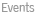Computational Mathematics and ApplicationsThe group aims at developing new techniques of mathematical modelling, mathematical analysis, and numerical algorithms for solving problems in applied areas. Currently, group members lead or are involved in multidisciplinary research projects and proposals covering a large domain of disciplinary such as Robotics, Biology, Physiscs and engineering applications. The mathematical analysis not only becomes the basis for defining the mathematical model, but is also essential to understand the properties of the model itself (e.g., the sensitivity to input data). Many of the above mentioned applications do require efficient numerical methods to determine relevant approximations of the solution. The numerical linear algebra is an essential tool in simulation and a part of the goup is dedicated on the design and analysis of algorithms for the computation of eigenvalues and singular values of large matrices, verys much in the spirit of the LAPACK and ScaLAPACK libraries. In general, mathematical models are governed by partial and ordinary differential equations and several teams of the group developing new numerical techniques based on the finite volume methods to provided accurate approximations of the complex and multi-physical problems. Specific lines of research of members of our group, in collaboration with colleagues from mathematics and applied areas, are:Numerical analysis of EDPs, mathematical modelling and scientific computingDynamic Neural Field theory and its application in neuroscience and cognitive roboticsWater use optimization in irrigation problemsApproximate solutions for piezoelectric problems for beams.Algorithms for structured matrices arising on digital image processingThe tridiagonal eigenproblemThe Jordan normal form factorizationEvaluation of an interior point filter line search method for solving nonlinear optimization problemsSpectral Computations and Integral Operator EquationsFinite volume method, high-order approximation, numerical fluid mechanicsAssessment of spatial correlations in a system of polarizable particles by measuring its optical response.

 © 2022 Universidade do Minho  - Legal Terms  - updated by CMATD.